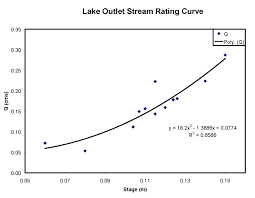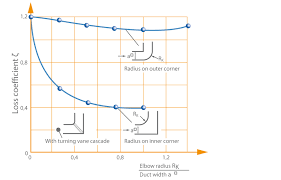## How to Calculate and Solve for Total Cross-Sectional Area of Gates | Design of Gating SystemThe image above represents total cross-sectional area of gates.

To compute for total cross-sectional area of gates, three essential parameters are needed and these parameters are Mass of the Casting (G), Specific Speed of Pouring (Ks) and Time of Pouring (t).

The formula for calculating total cross-sectional area of gates:

Fs = G / tKs

Where:

Fs = Total Cross-Sectional Area of Gates
G = Mass of the Casting
Ks = Specific Speed Of Pouring
t = Time of Pouring

Let’s solve an example;
Find the total cross-sectional area of gates when the mass of the casting is 10, specific speed of pouring is 18 and the time of pouring is 9.

This implies that;

G = Mass of the Casting = 10
Ks = Specific Speed Of Pouring = 18
t = Time of Pouring = 9

Fs = G / tKs
Fs = 10 / 9 x 18
Fs = 10 / 162
Fs = 0.0617

Therefore, the total cross-sectional area of gates is 0.0617 m2.

Calculating the Mass of the Casting when the Total Cross-Sectional Area of Gates, Specific Speed of Pouring and the Time of Pouring is Given.

G = Fs x tKs

Where:

G = Mass of the Casting
Fs = Total Cross-Sectional Area of Gates
Ks = Specific Speed Of Pouring
t = Time of Pouring

Let’s solve an example;
Find the mass of the casting when the total cross-sectional area of gates is 15, the specific speed of pouring is 7 and the time of pouring is 5.

This implies that;

Fs = Total Cross-Sectional Area of Gates = 15
Ks = Specific Speed Of Pouring = 7
t = Time of Pouring = 5

G = Fs x tKs
G = 15 x 5(7)
G = 15 x 35
G = 525

Therefore, the mass of the casting is 525 m.

## How to Calculate and Solve for Total Cross-Sectional Areas of Gates | Design of Gating System by a Hydraulic MethodThe image above represents total cross-sectional areas of gates.

To compute for total cross-sectional areas of gates, four essential parameters are needed and these parameters are mass of the casting (G), density of melt (ρ), pouring time (t) and pouring speed (v).

The formula for calculating total cross-sectional areas of gates:

Fg = G / ρtv

Where:

Fg = Total Cross-Sectional Area of Gates
G = Mass of the Casting
ρ = Density of Melt
t = Pouring Time
v = Pouring Speed

Let’s solve an example;
Find the total cross-sectional areas of gates when mass of the casting is 12, density of melt is 16, pouring time is 5 and pouring speed is 25.

This implies that;

G = Mass of the Casting = 12
ρ = Density of Melt = 16
t = Pouring Time = 5
v = Pouring Speed = 25

Fg = G / ρtv
Fg = 12 / 16 x 5 x 25
Fg = 12 / 2000
Fg = 0.006

Therefore, the total cross-sectional areas of gates is 0.006 m2.

Calculating the Mass of the Casting when the Total Cross-Sectional Areas of Gates, the Density of Melt, the Pouring Time and the Pouring Speed is Given.

G = Fg x ρtv

Where;

G = Mass of the Casting
Fg = Total Cross-Sectional Area of Gates
ρ = Density of Melt
t = Pouring Time
v = Pouring Speed

Let’s solve an example;
Find the mass of the casting when the total cross-sectional areas of gates is 32, the density of melt is 4, the pouring time is 10 and the pouring speed is 2.

This implies that;

Fg = Total Cross-Sectional Area of Gates = 32
ρ = Density of Melt = 4
t = Pouring Time  = 10
v = Pouring Speed = 2

G = Fg x ρtv
G = 32 x 4 x 10 x 2
G = 2560

Therefore, the mass of the Casting is 2560 m.